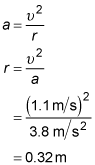##### Physics I: Practice Problems For DummiesUsing physics, you can find the centripetal acceleration of objects as they move in a circle. For example, you can calculate the acceleration of a ferry boat making a turn at a constant speed.

## Practice questions

1. A ferry boat makes a 180-degree turn in 12 minutes. The radius of the turn is 0.50 miles. What is its centripetal acceleration in meters per second squared during the turn?

There are 1,609 meters in 1 mile.

2. You twirl a lasso over your head at a constant angular speed of 3.8 radians per second. What is the centripetal acceleration in meters per second squared of the lasso's tip, 1.4 meters from your hand?

3. The documentation for your slot-car set says that the maximum centripetal acceleration the cars can withstand without being ejected from the track is 3.8 meters per second squared. You notice that the slot cars fly off the track if they exceed 1.1 meters per second. What is the radius in meters of the curve in the track?

The following are the answers to the practice questions:

1. 0.015 m/s2

First, convert the given quantities to meters and seconds. The time it takes to complete the turn is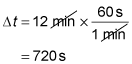The radius of the turn is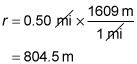The angular speed of the boat is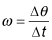where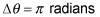because there are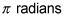in 180 degrees. Express the centripetal acceleration in terms of the angular speed using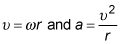Combining these two equations and the equation above for angular speed gives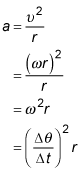Insert the known quantities: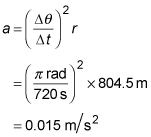2. 20 m/s2

The tangential speed of the tip of the lasso is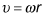where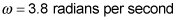is the angular speed and r = 1.4 meters is the radial distance from the center of the circle (that is, your hand) to the tip of the lasso. The centripetal acceleration is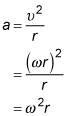Plug in the known quantities to find3. 0.32 m

The maximum centripetal acceleration is a = 3.8 meters per second squared, and the maximum speed at which the slot cars can go without flying off the track is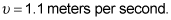Solve the equation for centripetal acceleration for the radius and insert these quantities. The result is